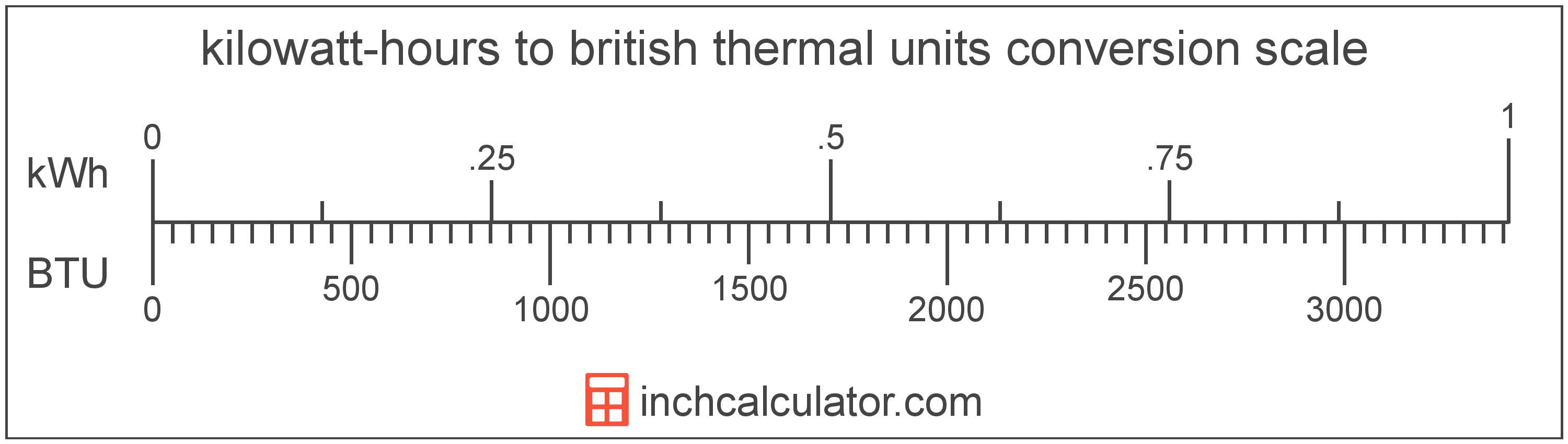# Kilowatt-Hours to British Thermal Units Converter

Enter the energy in kilowatt-hours below to get the value converted to british thermal units.

Results in British Thermal Units:1 kWh = 3,412.141633 BTU

Do you want to convert british thermal units to kilowatt-hours?

## How to Convert Kilowatt-Hours to British Thermal Units

To convert a measurement in kilowatt-hours to a measurement in british thermal units, multiply the energy by the following conversion ratio: 3,412.141633 british thermal units/kilowatt-hour.

Since one kilowatt-hour is equal to 3,412.141633 british thermal units, you can use this simple formula to convert:

british thermal units = kilowatt-hours × 3,412.141633

The energy in british thermal units is equal to the energy in kilowatt-hours multiplied by 3,412.141633.

For example, here's how to convert 5 kilowatt-hours to british thermal units using the formula above.
british thermal units = (5 kWh × 3,412.141633) = 17,060.708166 BTU### How Many British Thermal Units Are in a Kilowatt-Hour?

There are 3,412.141633 british thermal units in a kilowatt-hour, which is why we use this value in the formula above.

1 kWh = 3,412.141633 BTU

## What is a Kilowatt-Hour?

A kilowatt-hour is a measure of electrical energy equal to one kilowatt, or 1,000 watts, of power over a one hour period. Kilowatt-hours are a measure of electrical work performed over a period of time, and are often used as a way of measuring energy usage by electric companies.

Kilowatt-hours are usually abbreviated as kWh, although the formally adopted expression is kW·h. The abbreviation kW h is also sometimes used. For example, 1 kilowatt-hour can be written as 1 kWh, 1 kW·h, or 1 kW h.

In formal expressions, the centered dot (·) or space is used to separate units used to indicate multiplication in an expression and to avoid conflicting prefixes being misinterpreted as a unit symbol.

## What is a British Thermal Unit?

British thermal units are a measure of heat energy. Specifically, one british thermal unit is equal to the amount of heat energy required to increase the temperature of one pound of water one degree Fahrenheit. They are often used as a way to rate the energy heat producing appliances such furnaces, and to price heating fuels such as natural gas.

The british thermal unit is a US customary unit of energy. British thermal units can be abbreviated as BTU; for example, 1 british thermal unit can be written as 1 BTU.

## Kilowatt-Hour to British Thermal Unit Conversion Table

Table showing various kilowatt-hour measurements converted to british thermal units.
Kilowatt-hours British Thermal Units
0.001 kWh 3.4121 BTU
0.002 kWh 6.8243 BTU
0.003 kWh 10.24 BTU
0.004 kWh 13.65 BTU
0.005 kWh 17.06 BTU
0.006 kWh 20.47 BTU
0.007 kWh 23.88 BTU
0.008 kWh 27.3 BTU
0.009 kWh 30.71 BTU
0.01 kWh 34.12 BTU
0.02 kWh 68.24 BTU
0.03 kWh 102.36 BTU
0.04 kWh 136.49 BTU
0.05 kWh 170.61 BTU
0.06 kWh 204.73 BTU
0.07 kWh 238.85 BTU
0.08 kWh 272.97 BTU
0.09 kWh 307.09 BTU
0.1 kWh 341.21 BTU
0.2 kWh 682.43 BTU
0.3 kWh 1,024 BTU
0.4 kWh 1,365 BTU
0.5 kWh 1,706 BTU
0.6 kWh 2,047 BTU
0.7 kWh 2,388 BTU
0.8 kWh 2,730 BTU
0.9 kWh 3,071 BTU
1 kWh 3,412 BTU

## References

1. Bureau International des Poids et Mesures, The International System of Units (SI), 9th edition, 2019, https://www.bipm.org/documents/20126/41483022/SI-Brochure-9-EN.pdf